No TitleMaximum Intensity Estimation

Kerry A. Emanuel

Massachusetts Institute of Technology

The upper bound on tropical cyclone intensity is calculated using sea surface temperature and atmospheric thermodynamic profiles from the latest output of the Medium Range Forecasting (MRF) model from the National Centers for Environmental Prediction. The technique represents a generalization of that presented in Emanuel (1995; hereafter, E95) to the case of an open-cycle heat engine. The description presented by Emanuel (1995) has been further modified to account for dissipative heating, which had been neglected in earlier treatments. These modifications are described in Bister and Emanuel (1998). The input to the scheme at each grid point is the sea surface temperature and vertical profiles of pressure, temperature, and mixing ratio. (The FORTRAN subroutine is available from the author.)

For the purposes of this calculation, the work done against friction in the hurricane outflow is ignored. If the size of the storm is known, this work can be estimated, but it is usually very small unless the storm has an exceptionally large diameter (Emanuel, 1986). We also assume that at maximum intensity, the anticyclone at the storm top is fully developed (zero absolute vorticity) and that the gradient wind may be approximated by the cyclostrophic wind at the radius of maximum winds. When these conditions are satisfied, the thermal wind equation can be combined with an equation governing entropy in the boundary layer in regions near the eyewall where little entrainment of dry air through the top of the boundary layer occurs, resulting in: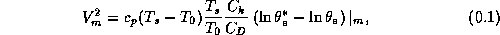where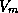is the maximum gradient wind speed,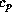is the heat capacity at constant pressure,is the ocean temperature,the mean outflow temperature,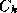the exchange coefficient for enthalpy,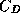the drag coefficient,the saturation equivalent potential temperature at the ocean surface, and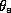the boundary layer equivalent potential temperature. The last factor in (1) is evaluated at the radius of maximum winds. This expression is the dimensional equivalent of Eq. (13) of E95, and can also be writtenwhereis the entropy () and the closed cycle is for a parcel beginning at the ambient boundary layer value ofand winding up at saturation. Entropy is acquired at temperatureand exported at temperature. It is important to note that (2) does not imply that air parcels actually become saturated at sea level under the eyewall.

The integral in (2) is also equal to the convective available potential energy (see Emanuel, 1994, Eq. 6.4.2). Thus (1) can be written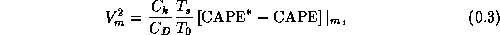where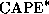is the convective available potential energy of air lifted from saturation at sea level in reference to the environmental sounding, and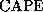is that of boundary layer air. Both quantities are evaluated near the radius of maximum wind.

To evaluate (3) it is first necessary to determine the surface pressure at the radius of maximum winds, needed to calculate the saturation mixing ratio necessary for. To do so, we use a combination of gradient wind balance and thermal wind balance in the outer region of the hurricane. This is equation (6) of E95, whose dimensional equivalent (assuming cyclostrophic balance at the radius of maximum winds) is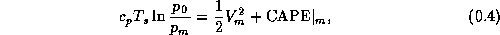where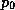is the ambient surface pressure and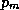is the surface pressure at the radius of maximum winds.

To calculate, the mixing ratio and temperature of the boundary layer under the eyewall is needed. Following E95, we assume that the surface temperature isand that the relative humidity is constant from the outer region to the outer edge of the eyewall. Since the pressure under the eyewall is lower than ambient, this entails a small inward increase in mixing ratio. (Experiments assuming constant mixing ratio rather than constant relative humidity yield only slightly different results.) For this reason,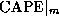is a little larger than theof ambient boundary layer air. The assumption of constant relative humidity in the outer region boundary layer is well supported by numerical simulations.

Given a value of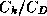, the sea surface temperature, and an ambient profile of virtual temperature, (3) and (4) constitute closed relations forand.is calculated by a reversible adiabatic parcel lifting algorithm. Owing to the pressure dependence ofand, (3) and (4) must be solved iteratively. This always converges unless one is in the ``hypercane'' regime.

Lastly, we estimate the central pressure as in E95 by assuming that the flow inside the radius of maximum winds is in solid body rotation and cyclostrophic balance. This yields the relationwhere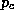is the central surface pressure.

In the output of the subroutine used to calculateand, the latter has been reduced by 20% to reflect the typical relationship between gradient winds and actual surface winds.

References

Emanuel, K. A., 1986: An air-sea interaction theory for tropical cyclones. Part I. J. Atmos. Sci., 42, 586-604.

Emanuel, K. A., 1994: Atmospheric Convection. New York, Oxford Univ. Press, 580 pp.

Emanuel, K. A., 1995: Sensitivity of tropical cyclones to surface exchange coefficients and a revised steady-state model incorporating eye dynamics. J. Atmos. Sci., 52, 3969-3976.